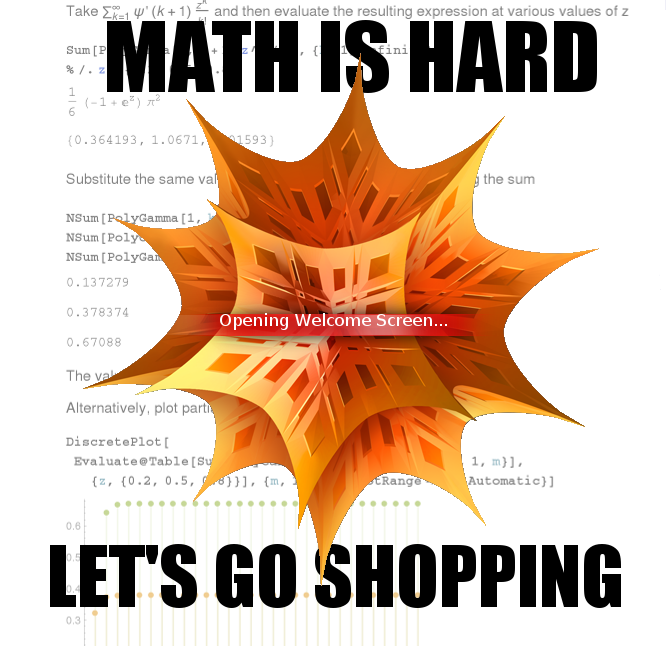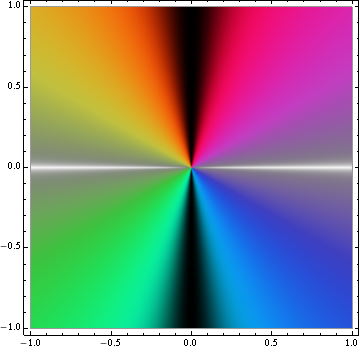1. 2014
Nov
20

## Another Mathematica bugMath is hard.

Not for Barbie, but for Mathematica.

I ran into a weird Mathematica bug while trying to evaluate the sum

$$\sum_{k=1}^{\infty} \biggl[-\frac{\pi^2}{6} + \psi'(k + 1) + H_k^2\biggr]\frac{z^k}{k!}$$

Split this into three parts. The first one is the well-known expansion of the exponential function

$$-\frac{\pi^2}{6}\sum_{k=1}^{\infty} \frac{z^k}{k!} = -\frac{\pi^2}{6}(e^z - 1)$$

The second is not the well-known expansion of the exponential function.

$$\sum_{k=1}^{\infty} \psi'(k + 1)\frac{z^k}{k!} \neq \frac{\pi^2}{6}(e^z - 1)$$

Obviously not, in fact, since if two power series are equal, $$\sum_i a_n z^n = \sum_i b_n z^n$$, for an infinite number of points, each of their coefficients have to be equal: $$\forall n,\ a_n = b_n$$. (You can show this by taking the difference of the two sides and plugging in a bunch of different values of $$z$$.)

I guess Mathematica doesn’t know that.

In = Sum[PolyGamma[1, k + 1] z^k/k!, {k, 1, Infinity}]
Out = 1/6(-1 + E^z)Pi^2


I had my hopes up for …

2. 2012
Oct
03

## Your computer algebra system is out to get you!

OK, maybe it’s not what you think, the CAS‘s of the world are not going to rise up and enslave us :-P (Not yet, anyway.) But it is always a good idea to view their results with a healthy dose of skepticism, because like any computer program, they have bugs, and every once in a while one can actually mess up your calculation.

Here’s my example:

$$\int_0^{2\pi} \cos x\,e^{ik\cos(x - y)}\udc x$$

Plug this into Mathematica 8.0 and it will tell you that the integral is equal to $$2\pi i J_1(k)$$, where $$J_1$$ is a Bessel function of the first kind:

In  := Integrate[Cos[x] Exp[I k Cos[x - y]], {x, 0, 2 Pi}]
Out := ConditionalExpression[2 I \[Pi] BesselJ[1, k], k \[Element] Reals]


But it’s not! If you shift the integration variable $$x\to x + y$$, Mathematica tips its hand and tells you the real answer, $$2\pi i J_1(k)\cos y$$:

In  := Integrate[Cos[x + y] Exp[I k Cos[x]], {x, 0, 2 Pi}]
Out := 2 Pi I BesselJ[1, k] Cos[y]


You can also do …

3. 2012
Feb
10

## Symbolic operators in Mathematica

This originally arose as a post I was going to make on Mathematica Stack Exchange, but the question wasn’t well defined enough for Stack Exchange’s Q&A format. So I’m reposting it here. The list is incomplete right now but I’ll keep adding to it as I have time.

Each of the operators below is a shortened notation for some piece of Mathematica syntax or for a Mathematica function. After a short description of each operator, I list one or two usage example along with a way of representing the same thing using different syntax.

• @ is the prefix notation for function application. This applies the single-argument function whose name precedes it to the expression that follows it.

func @ expr
func[expr]


(remember, that’s a usage example and an equivalent way of writing the same thing using different notation)

• // is the postfix notation for function application. This applies the single-argument function whose name follows it to the expression that precedes it.

expr // func
func[expr]

• ~ is the infix notation for function application. This has a slightly unusual usage pattern: you have to use it twice, in the form arg1 ~ func ~ arg2, and it applies the two-argument …

4. 2011
Oct
31

## Running Mathematica notebooks in batch mode

If you’re like me, you’re used to thinking of Mathematica as an interactive calculator, where you type in an expression and Mathematica evaluates it and spits out the result. But the Mathematica system actually incorporates a whole programming language, and as you might expect of any programming language, it’s possible to write Mathematica programs (scripts) that run non-interactively.

In order to write a Mathematica script, you create a text file which contains the expressions you want to evaluate (in normal programming terminology, the statements to execute). The conventional file extension for Mathematica scripts is .m. Each expression that you would normally type into the Mathematica window, you type into the script file, one per line. (Line breaks are allowed within expressions under certain circumstances.) You can then run the script using

math -script filename.m


(on Linux). The evaluated expressions, which Mathematica would normally show you right below where you typed in the input, are printed to standard output, so I recommend ending lines with a semicolon (which suppresses the output) by default. Only leave the semicolon off for the results you actually care about.

Keep in mind that a Mathematica script file is not what you …

5. 2011
Jun
17

## Bound local variables in Mathematica

When writing a computer program in a language that allows function definitions inside code blocks, it’s pretty common to create a function that references some variable in an enclosing scope. And sometimes you want to delete that variable without making it inaccessible to the function. In Mathematica, you can use Module to do this:

a = 4;
f[x_] = Module[{b=a}, If[x>0,x+b,0]];


Since I used a regular assignment = to define f[x_] rather than delayed assignment :=, this stores the value of a in a “local” variable named something like b\$120938, so even after I run

Clear[a];


f[x] still adds four to $$x$$, so, for example, f would return 5.

In contrast, if I use Block, Mathematica won’t store the value of a.

a = 4;
f[x_] = Module[{b=a}, If[x>0,x+b,0]];
Clear[a];


After this code snippet f would just return 1+b. (For some reason Mathematica doesn’t seem to recognize that b has a value of a inside the If statement… I’m not sure if this is intentional behavior.)

6. 2011
Mar
28

## Two complex color functionsIn the process of graphing some complex valued functions, I figured out a couple of neat coloring schemes that I thought might be worth sharing. Both schemes share the same hue and saturation functions:

$$H = \arg{z}-\frac{\pi}{2}$$
$$S = \abs{\frac{\imag{z}}{z}}$$

They differ in the third component, though. The one which I will arbitrarily designate “color scheme 1” (because I like it better) is defined in HSL color space, and has a luminosity function of

$$L = \frac{1}{2}\biggl[1 - 2^{20}\abs{S - \frac{1}{2}}^{20}\sgn\biggl(S - \frac{1}{2}\biggr)\biggr]$$

where $$S$$ is the saturation value. This maps all real or nearly real numbers to white, all imaginary or nearly imaginary numbers to black, and other complex numbers to varying hues depending on their phase.

The other one, “color scheme 2,” has a value (brightness) function of

$$V = 1 - \frac{S}{4}$$

This maps all real or nearly real numbers to white, and all other numbers to varying hues, again depending on their phase. Positive imaginary numbers are red, negative imaginary numbers are cyan.

Note that both functions are undefined at $$z = 0$$. If you implement these you …